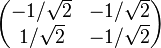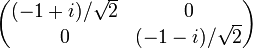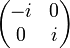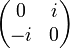# Faithful irreducible representation of dihedral group:D16

Jump to: navigation, search
This article describes a particular irreducible linear representation for the following group: dihedral group:D16. The representation is unique up to equivalence of linear representations and is irreducible, at least over its original field of definition in characteristic zero. The representation may also be definable over other characteristics by reducing the matrices modulo that characteristic, though it may behave somewhat differently in these characteristics.
For more on the linear representation theory of the group, see linear representation theory of dihedral group:D16.

Here,$G$ is the dihedral group:D16, the dihedral group of degree 8and order 16, given by the presentation:$G = D_{16} := \langle a,x \mid a^8 = x^2 = e, xax^{-1} = a^{-1} \rangle$

## Summary

This is actually a collection of two faithful irreducible two-dimensional representations of the group$D_{16}$, which form a single orbit under the action of the automorphism group, and also form a single orbit under the action of Galois automorphisms in the field of realization (at least in characteristic zero).

Item Value
degree of representation (dimension of space on which it is realized, or order of matrices) 2
Schur index value of representation 1
Kernel of representation trivial subgroup, i.e., it is a faithful linear representation
Quotient on which it descends to a faithful linear representation dihedral group:D16
Set of character values$\{ 2, 0, -2, \sqrt{2}, -\sqrt{2} \}$ (interpreted/reduced over the ring/field --$\sqrt{2}$ is a square root of 2
Characteristic zero: Ring generated --$\mathbb{Z}[\sqrt{2}] = \mathbb{Z}[t]/(t^2 - 2)$, Ideal within ring generated -- Principal ideal generated by$\sqrt{2}$, Field generated --$\mathbb{Q}(\sqrt{2}) = \mathbb{Q}[t]/(t^2 - 2)$
Rings of realization The representations can be realized precisely rings containing a square root of 2.
Fields of realization The representations can be realized precisely fields containing a square root of 2.
For a finite field with$q$ elements,$q$, this is equivalent to$q \equiv 1 \pmod 8$
Minimal field of realization In characteristic zero:$\mathbb{Q}(\sqrt{2}) = \mathbb{Q}[t]/(t^2 - 2)$
In characteristic$p \equiv \pm 1 \pmod 8$: prime field$\mathbb{F}_p$
In characteristic$p \equiv \pm 3 \pmod 8$: field$\mathbb{F}_{p^2}$, quadratic extension of$\mathbb{F}_p$

## Representation table

There are two such representations, and they are related by the group automorphism$a \mapsto a^3, x \mapsto x$, and also by the Galois automorphism$\sqrt{2} \mapsto -\sqrt{2}$ for the extension$\mathbb{Q}(\sqrt{2})$ over$\mathbb{Q}$.

We give each of these representations in three forms. One is a representation as orthogonal matrices (with the generator$a$ mapping to rotation by an odd multiple of$\pi/4$ and$x$ mapping to a reflection), and this representation is realized over the ring$\mathbb{Z}[1/\sqrt{2}]$. The second is as complex unitary matrices. The third epresentation is realized over the smaller subring$\mathbb{Z}[\sqrt{2}]$ but the matrices are no longer orthogonal matrices.

Here is the first representation in all three forms:

The table below is incomplete, it has only 11 of the 16 elements, more will be added later

Element Matrix as real orthogonal Matrix as complex unitary Matrix as real, non-orthogonal, in$\mathbb{Z}[\sqrt{2}]$ Characteristic polynomial Minimal polynomial Trace, character value Determinant$e$$\begin{pmatrix} 1 & 0 \\ 0 & 1 \\\end{pmatrix}$$\begin{pmatrix} 1 & 0 \\ 0 & 1 \\\end{pmatrix}$$\begin{pmatrix} 1 & 0 \\ 0 & 1 \\\end{pmatrix}$$(t - 1)^2 = t^2 - 2t + 1$$t - 1$ 2 1$a$$\begin{pmatrix} 1/\sqrt{2} & -1/\sqrt{2} \\ 1/\sqrt{2} & 1/\sqrt{2} \\\end{pmatrix}$$\begin{pmatrix} (1 + i)/\sqrt{2} & 0 \\ 0 & (1 - i)/\sqrt{2}\\\end{pmatrix}$$\begin{pmatrix} 0 & -1 \\ 1 & \sqrt{2} \\\end{pmatrix}$$t^2 - \sqrt{2} t + 1$$t^2 - \sqrt{2} t + 1$$\sqrt{2}$ 1$a^2$$\begin{pmatrix} 0 & -1 \\ 1 & 0 \\\end{pmatrix}$$\begin{pmatrix} i & 0 \\ 0 & -i \\\end{pmatrix}$$\begin{pmatrix} -1 & -\sqrt{2} \\ \sqrt{2} & 1 \\\end{pmatrix}$$t^2 + 1$$t^2 + 1$ 0 1$a^3$$\begin{pmatrix} -1/\sqrt{2} & -1/\sqrt{2} \\ 1/\sqrt{2} & -1/\sqrt{2} \\\end{pmatrix}$$\begin{pmatrix} (-1 + i)/\sqrt{2} & 0 \\ 0 & (-1-i)/\sqrt{2}\\\end{pmatrix}$$\begin{pmatrix} -\sqrt{2} & -1 \\ 1 & 0 \\\end{pmatrix}$$t^2 + \sqrt{2}t + 1$$t^2 + \sqrt{2}t + 1$$-\sqrt{2}$ 1$a^4$$\begin{pmatrix} -1 & 0 \\ 0 & -1 \\\end{pmatrix}$$\begin{pmatrix} -1 & 0 \\ 0 & -1 \\\end{pmatrix}$$\begin{pmatrix} -1 & 0 \\ 0 & -1 \\\end{pmatrix}$$(t + 1)^2 = t^2 + 2t + 1$$t + 1$ -2 1$a^5$$\begin{pmatrix} -1/\sqrt{2} & 1/\sqrt{2} \\ -1/\sqrt{2} & -1/\sqrt{2} \\\end{pmatrix}$$\begin{pmatrix} (-1 - i)/\sqrt{2} & 0 \\ 0 & (-1 + i)/\sqrt{2} \\\end{pmatrix}$$\begin{pmatrix} 0 & 1 \\ -1 & -\sqrt{2} \\\end{pmatrix}$$t^2 + \sqrt{2}t + 1$$t^2 + \sqrt{2}t + 1$$-\sqrt{2}$ 1$a^6$$\begin{pmatrix} 0 & 1 \\ -1 & 0 \\\end{pmatrix}$$\begin{pmatrix} -i & 0 \\ 0 & i \\\end{pmatrix}$$\begin{pmatrix} 1 & \sqrt{2} \\ -\sqrt{2} & -1 \\\end{pmatrix}$$t^2 + 1$$t^2 + 1$ 0 1$a^7$$\begin{pmatrix} 1/\sqrt{2} & 1/\sqrt{2} \\ -1/\sqrt{2} & 1/\sqrt{2} \\\end{pmatrix}$$\begin{pmatrix} (1 - i)/\sqrt{2} & 0 \\ 0 & (1 + i)/\sqrt{2}\\\end{pmatrix}$$\begin{pmatrix} \sqrt{2} & 1 \\ -1 & 0 \\\end{pmatrix}$$t^2 - \sqrt{2}t + 1$$t^2 - \sqrt{2}t + 1$$\sqrt{2}$ 1$x$$\begin{pmatrix} 1 & 0 \\ 0 & -1 \end{pmatrix}$$\begin{pmatrix} 0 & 1 \\ 1 & 0 \\\end{pmatrix}$$\begin{pmatrix}0 & 1 \\ 1 & 0 \\\end{pmatrix}$$t^2 - 1$$t^2 - 1$ 0 -1$ax$$\begin{pmatrix} 1/\sqrt{2} & 1/\sqrt{2} \\ 1/\sqrt{2} & -1/\sqrt{2} \\\end{pmatrix}$$\begin{pmatrix} 0 & (1 + i)/\sqrt{2} \\ (1 - i)/\sqrt{2} & 0 \\\end{pmatrix}$$\begin{pmatrix} -1 & 0 \\ \sqrt{2} & 1 \\\end{pmatrix}$$t^2 - 1$$t^2 - 1$ 0 -1$a^2x$$\begin{pmatrix} 0 & 1 \\ 1 & 0 \\\end{pmatrix}$$\begin{pmatrix}0 & i \\ -i & 0 \\\end{pmatrix}$$\begin{pmatrix} -\sqrt{2} & -1 \\ 1 & \sqrt{2} \\\end{pmatrix}$$t^2 - 1$$t^2 - 1$ 0 -1